Mathematics
Geometry Proofs List
52.8k views

 1 Introduction 2 Geometry Proofs List 3 How to solve geometry proofs? 4 Summary 5 FAQs

29 October 2020

## Introduction

Geometry is the study of visualizations. Perceiving what objects/ images mean/ signify is a major part of the work in this area of study.

Children often struggle with geometry since it is a jump from the basic concepts of algebra into something more abstract and unique. And because it is so different from what children have learned before, the art of teaching it should vary too.Unfortunately, the school curriculum does not account for that and goes on teaching in the same format. The small inconvenience of not being able to understand a concept stems from something stronger and severe as children grow - the fear of geometry & math.

If your child struggles with geometry, it could be for the following reasons:

• Unable to understand & apply the vocabulary to decode the problem.
• Can’t see or imagine all of the pieces that go into making up the Geometry problem.
• Struggle with the Algebra skills involved in doing Geometry

But even if learning geometry comes easy to them, one thing that the whiz kids find tough is with proofs!

And what better way to help sort these proofs out than a geometry proofs list compiling the list of geometry proofs and references to geometry proofs.Geometry proofs are what math actually is. To put it simply- they're the explanation, and everything else follows from them. This means they're the most important part of the whole field by a very large measure, but they're generally going to be more difficult than anything else.

We are going to share an important geometry proofs list, that your children should be familiar with. And also explain how to solve geometry proofs.

Worry not, Cuemath has a way around that to ensure every child not only learns proofs and applies them, but also loves the process of learning them.

We have attached corresponding topic links in the geometry proofs list and statements mentioned for a deeper understanding of each.

## Geometry Proofs List

If your children have been learning geometry, they would be familiar with the basic proofs like the definition of an isosceles triangle, Isosceles Triangle Theorem, Perpendicular, acute & obtuse triangles, Right angles, ASA, SAS, AAS & SSS triangles.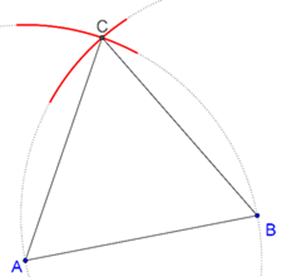All of these proofs, like anything else, require a lot of practice. They're inherently different from solving problems because you already know the result and are solving for it. All kids need to do is manipulate the logic and structures after understanding how to solve these geometry proofs.

Here are some geometric proofs they will learn over the course of their studies:

### Parallel Lines

If any two lines in the same plane do not intersect, then the lines are said to be parallel.

Certain angles like vertically opposite angles and alternate angles are equal while others are supplementing to each other.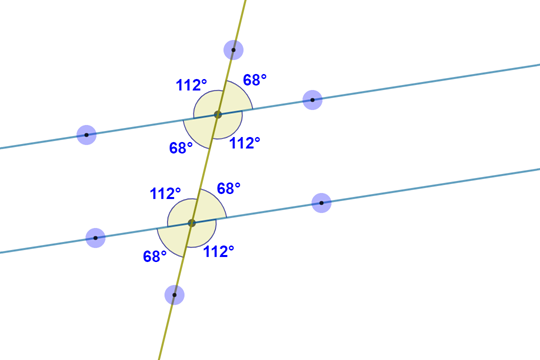### Corresponding Angles

If two parallel lines are cut by a transversal, then the pairs of corresponding angles are congruent.

### Vertical Angles

States, “If two non-adjacent angles are created by intersecting lines, then those angles are known as vertical angles.”

### The Definition of Isosceles Triangle

Says that “If a triangle is isosceles then TWO or more sides are congruent.”

### Isosceles Triangle Theorem

Says that “If a triangle is isosceles, then its BASE ANGLES are congruent.” This applies to the above point that you have already learned.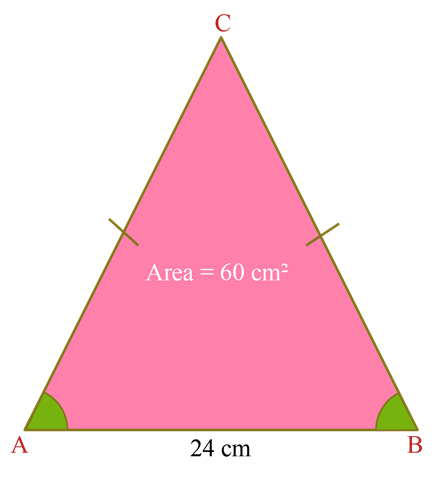### Acute Triangle/ Obtuse Triangle

Says that “If a triangle is an acute triangle, then all of its angles are less than 90 degrees.”

And, “If a triangle is an obtuse triangle, then one of its angles is greater than 180 degrees.”

### Perpendicular

States “If two lines, rays, segments or planes are perpendicular, then they form right angles (as many as four of them).”

### Right Angle/ Acute Angle/ Obtuse Angle

States, “If an angle is a right angle, then the angle must EQUAL 90 degrees.”

“If an angle is an acute angle, then the angle must be less than 90 degrees.”

“If an angle is an obtuse angle, then the angle must be greater than 90 degrees.”

### Congruency of Triangles: ASA, SSS, SAS, AAS

• ASA

States, if the two angles and the side included between them of one triangle are equal to the two corresponding angles and the side included between them of another triangle, the two triangles are congruent.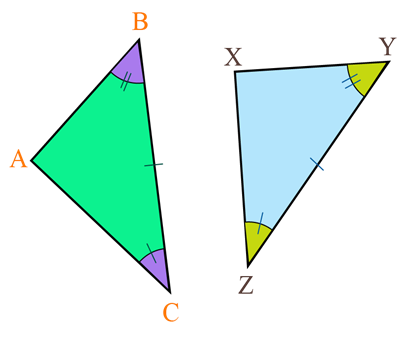• SSS

states, if the three sides of one triangle are equal to the three corresponding sides of another triangle, the two triangles are congruent.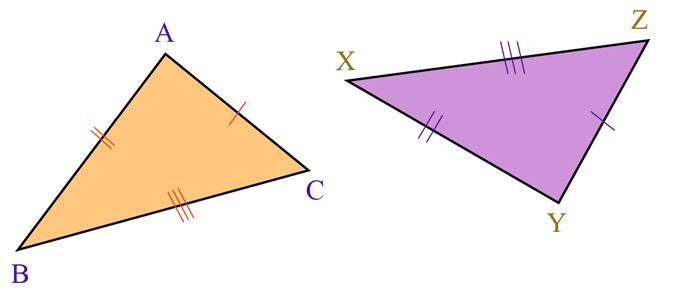• SAS

says that “If two sides and an included angle of one triangle are congruent to two corresponding sides and an included angle of another triangle, then the triangles are congruent.”

• RHS

States, if the hypotenuse and side of one right-angled triangle are equal to the hypotenuse and the corresponding side of another right-angled triangle, the two triangles are congruent.

• AAS

says that “If two angles and non-included sides of one triangle are congruent to two angles and a non-included side of another triangle, then the triangles are congruent.”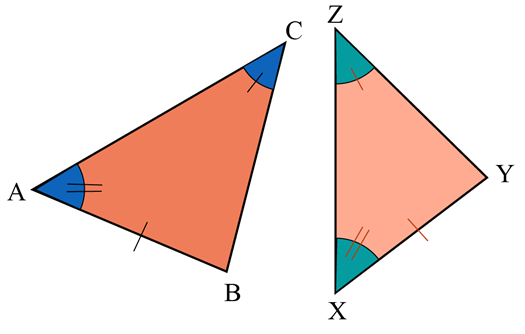### Similarity

Angle-Angle (AA) Similarity

If the three angles of one triangle are respectively equal to the three angles of the other, then the two triangles will be similar

SSS for Similarity

if the three sets of corresponding sides of two triangles are in proportion, the triangles are similar.

SAS for Similarity

If an angle of one triangle is congruent to the corresponding angle of another triangle and the lengths of the sides including these angles are in proportion, the triangles are similar

### CPCTC

States that “If you use ASA, SSS, SAS, or AAS to prove that two triangles are congruent, then all other corresponding parts (sides & angles) of the congruent triangles are going to be congruent.”

### Reflexive Property

States, “something is congruent to itself.”

used when we do part + part = whole (for either sides or angles).

### Interior angles of Triangle

Adding up all the interior angles of a triangle gives 180º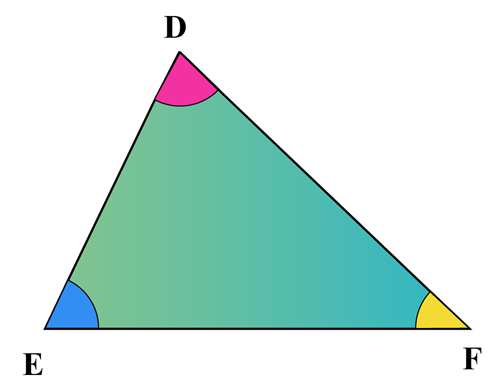### Segment Bisector

States “If a segment, ray, line or plane is a segment bisector, then it divides a segment into TWO equal parts.”

### Definition of Altitude

States, “If a segment is an altitude, then it is a segment originating from one of the vertices of a triangle and its perpendicular to an opposite side.”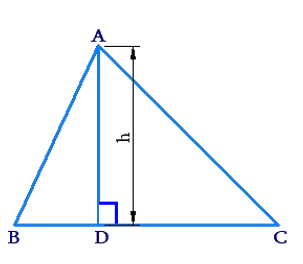### Exterior Angle

The measure of an exterior angle of a triangle is equal to the sum of the measures of the two non-adjacent interior angles and the value is greater than either non-adjacent interior angle.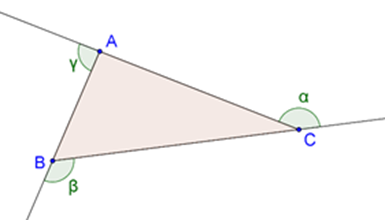### Circle Proofs used

• The radius of a circle is always perpendicular to a chord, bisects the chord and the arc.

• A tangent dropped to a circle, is perpendicular to the radius made at the point of tangency.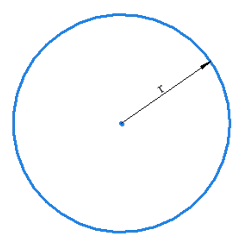• Tangent segments from a single point to a circle at different points are equal.

• An angle inscribed in a semi-circle or half-circle is a right angle.

This was the important geometry proofs list.

This list of geometry proofs form the base to other proofs and theorems that your child will learn. It is essential for children to learn & pay attention to the general styles of proofs so that they would be able to apply it to other problems.

Once they get thorough with the geometry proofs list, they would get an intuition for how different structures act and interact and what strategies might be best to apply..this way they won't even find geometry hard, and will be able to solve the complete list of geometry proofs.

## How to solve geometry proofs?

Now that we know the importance of being thorough with the geometry proofs list, here is how you can get your children to tackle the list of geometry poofs.

### 1. Plan it out

Einstein once said that if he had 60 min to solve a problem, he would spend 58 minutes defining the problem statement. Defining the problem statement helps with planning, and as experts say, planning is the first step to tackling a problem.Familiarize your children with the importance of planning right. Teach them to start by writing out the problem in plain English, with no mathematical jargon. Solving Geometry proofs just got a lot simpler.

### 2. Look for lengths, angles, and keep CPCTC in mind

All the geometry concepts your child has learned would come to life here. They could start by allocating lengths for segments or measures for angles & look for congruent triangles.

### 3. Parallel Lines can be a lifesaver

This is an old trick that you would be familiar with as well. Any parallel lines in the proof’s diagram mean that you would use one of the parallel-line theorems.

Pass on this wisdom to help your children solve geometry proofs given in the geometry proofs list.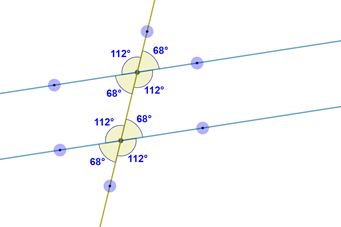### 4. If-then logic is always gold

Another one from the bag of tricks, ‘all the ideas in the if clause appears in the statement column somewhere above the line you‘re checking’.

This theory also helps to figure out what reason to use in the first place. This can work on any one of the theorems in the geometry proofs list!

### 5. If you get stuck, work backward

Jump to the end of the proof and start making guesses about the reasons for that conclusion. You can almost always figure out the way by using the if-then logic to reach the previous statement (and so on).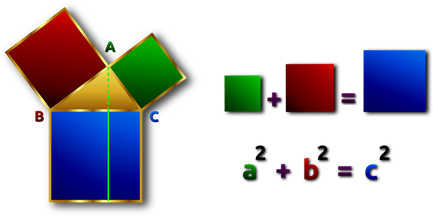/em>

## Summary

Geometry proofs don't have to be hard for the kids, but we hope that with the right guidance, they will be familiar with how to solve geometry proofs. The geometry proofs list, along with tips on how to solve geometry proofs can be a good start to train your child and get them to love geometry.

Written by Sonia Motwani